# CLIJ2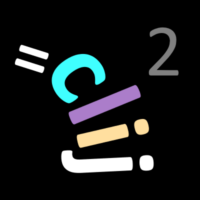GPU accelerated image processing for everyone

CLIJ2 home

# Process multi-channel time-lapse data

This notebook demonstrates how image data can be processed that has multiple channels and time points.

Source

Let’s start by cleaaning up and opening an example dataset.

```// clean up first
run("Close All");
run("Clear Results");

// open a the Mitosis example image
open("http://imagej.nih.gov/ij/images/Spindly-GFP.zip");
```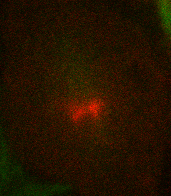We now initialize the GPU and push the image as explained in the basics of clij tutorial.

```run("CLIJ2 Macro Extensions", "cl_device=");
Ext.CLIJ2_clear();
```

## Processing multiple channels

Pushing an image to GPU means in this context, that only the currently selected channel and time point are pushed.

```// push current Z-stack image to GPU memory
input = getTitle();
Stack.setChannel(1);
Ext.CLIJ2_pushCurrentZStack(input);
```

We can then process the channel, e.g. by projecting it in Z:

```Ext.CLIJ2_maximumZProjection(input, max_projection_c1);
Ext.CLIJ2_pull(max_projection_c1);
run("Red");
```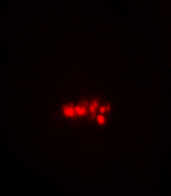Afterwards, we can do the same with the other channel:

```selectWindow(input);
Stack.setChannel(2);
Ext.CLIJ2_pushCurrentZStack(input);
Ext.CLIJ2_maximumZProjection(input, max_projection_c2);
Ext.CLIJ2_pull(max_projection_c2);
run("Green");
```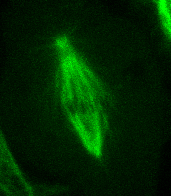Channels can then be merged using ImageJs functionality

```run("Merge Channels...", "c1=" + max_projection_c1 + " c2=" + max_projection_c2 + " create");
```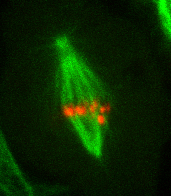## Processing time-lapse data

We can apply the same procedure to the whole time-lapse using a for-loop:

```
selectWindow(input);
// find out how many frames the time-lapse has
getDimensions(_, _, _, _, frames);

// loop over time points
for (t = 0; t < frames; t += 5) {
// move forward in time in the dataset
selectWindow(input);
Stack.setFrame(t + 1); // ImageJ's frame-counter is 1-based

// process channel 1
Stack.setChannel(1);
Ext.CLIJ2_pushCurrentZStack(input);
Ext.CLIJ2_maximumZProjection(input, max_projection_c1);
Ext.CLIJ2_pull(max_projection_c1);
run("Red");

// process channel 2
selectWindow(input);
Stack.setChannel(2);
Ext.CLIJ2_pushCurrentZStack(input);
Ext.CLIJ2_maximumZProjection(input, max_projection_c2);
Ext.CLIJ2_pull(max_projection_c2);
run("Green");

// merge channels
run("Merge Channels...", "c1=" + max_projection_c1 + " c2=" + max_projection_c2 + " create");
}
```

Depending on how large data is, you may not want to show all results on screen. Instead, consider saving results of a 3D stack:

```Ext.CLIJ2_saveAsTIF(max_projection_c2, "max_projection_c2_f" + t + ".tif");

```

Clean up by the end

```Ext.CLIJ2_clear();

```